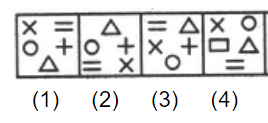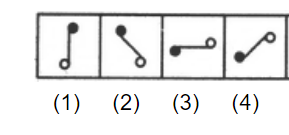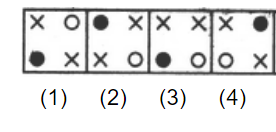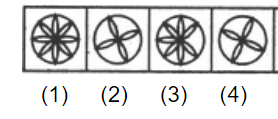# E-PolyLearning

 16. Choose the figure which is different from the rest.a. 1 b. 2 c. 3 d. 4

 17. Choose the figure which is different from the rest.a. 1 b. 2 c. 3 d. 4
 18. Choose the figure which is different from the rest.a. 1 b. 2 c. 3 d. 4
 19. Choose the figure which is different from the rest.a. 1 b. 2 c. 3 d. 4
 20. Choose the figure which is different from the rest.a. 1 b. 2 c. 3 d. 4